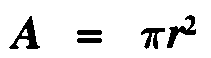#### Measurement Practice Problems

1. Complete the following table

 SYMBOL Fundamental Quantity Capacity Unit Preferred Metric Unit of Density Units of derived  quantities m length m2 area m3 cubic volume mL volume capacity volume L volume capacity volume g mass kg g/L density kg/m3 preferred density unit density

2. a) Calculate the area of a building lot 200 m long by 450 m deep.

b) Express the area in m2 as well as in hectares

c) if the price of land is \$2000 per hectare, wat is the cost of buying the lot

Solution:

Area = length X width

a)  A =   l  X  w
=  200 m  x  450 m
=  90 000 m2

b) In hectares (ha)

1 ha = 10 000 m  Therefore 90 000 m2 = 90 ha

c) Price of land at \$2 000/ha

90 ha  X 2 000 \$/ha  =  \$ 180 000

3.  How big is a hole (the area of the hole) vpriduced by a drill 1.8 cm diameter drill bit ?

Area of  Circle (drill bit produces circular holes) is given byGiven:   diameter = 1.8 cm
radius = diameter/2 = 0.9 cm

A = 3.14 X (0.9) 2  = 3.14 * 0.9 * 0.9

= 2.54 cm

4 a) A  transparent liquid inan industrual drum has a mass of m = 3000 kg.

The mass of the container (drum) is 500 kg and a volume of 2.5 m

What is the density of this liquidand what is this liquid made of?

Solution: Density = mass/Volume

D = m / V

Given ;  Net mass of liquid  3000 kg - 500 kg = 2500 kg      V = 2.5 m

D =   2500 kg / 2.5 m3   =   1000 kg / m3

b) The material is most likely water.  We can use a density table to look up the substance according to its density.

5. A block whose dimensions  in meters (m) are:
l = 0.50 m      length
w = 0.20 m    width
h =  0.15 m    height (or depth)

has a mass of 127.5 kg

m =127.5 kg    mass

a) Find the Volume of the block

V = l  X w X h
= 0.5 m X 0.20 m X 0.15 m  = 0. 015 m3

b) Find the Density of the block

D = m / V
= 127.5 kg / 0.015 m3
=   8 500 kg / m3

d) From a density table we can see that the metal is Brass

6. An irregularly shaped rock with mass 660 g is placed in a graduated cylinder containing 300 mL of water before the rock is slowly lowered in the cylinder.
With the rock in the cylinder, the volume of the water in it rose to 420 mL.  Find the density of the rock.

Given:
mass of rock,        m  =    660 g
volume of rock,    V  =   120 mL (the same as the water displaced)

a) 1 cm3 =1 mL    Therefore 120 mL = 120 cm3

b) D = m / V
= 660 g / 120 cm3
The density of the rock is  =  5.5 g / cm3